# Parser Expression Grammars

by

#### Gavin Wraith

24/04/07

RiscLua's lpeg library not only finds patterns in text, but lets you perform actions as the text is scanned. We will leave aside for the moment how to make patterns do things, and concentrate first on the raw notion of a pattern in a piece of text.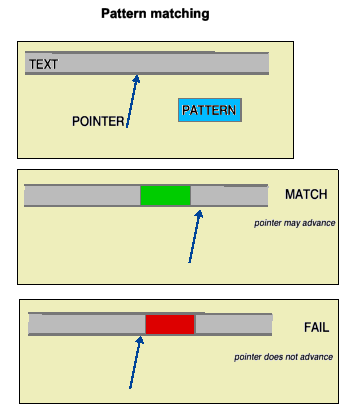Here we have a diagram depicting three elements: text, a pointer to a character in the text, and a pattern. The action of matching the pattern to the text, starting from the pointer, may have two sorts of outcome: MATCH - the pattern exists at the given position. The pointer may be advanced to the next piece of text. FAIL - the pattern fails at the given position. The pointer stays where it is. There may be different degrees of matching, and different reasons for failure, according to the complexity of the pattern. The pointer can only move forwards. Now we consider the ways in which patterns can be combined. We may concatenate patterns. This operation is denoted by multiplication in the lpeg notation. It is not commutative, of course. When the pattern A*B is matched, first A is tested at the current pointer. If A fails, A*B fails and B does not get tested at all. If A matches, B is tested at the new pointer position. If B fails we have a partial match. If B matches then A*B matches and the pointer is updated again. The multiplicative notation is extended to exponentiation. If n is a nonnegative integer then A^n matches if there are at least n consecutive instances of text matching A. Note that A^0 matches even when there is no text matching A; that is, the empty string matches A^0. The expression A^(-n) matches if there are at most n consecutive instances of text matching A. In particular, A^(-1) is matched by an empty string or by a string matching A.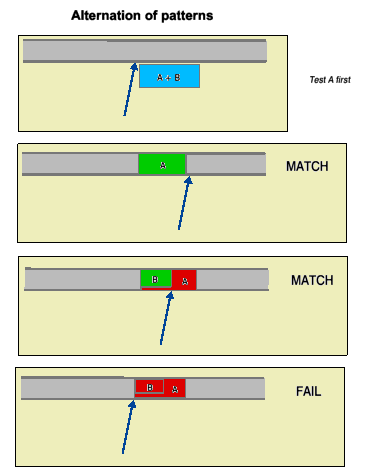Alternation of patterns is denoted by addition. The pattern A+B is matched first by testing A at the pointer. If A matches then A+B matches, B is not tested, and the pointer is advanced past the text matching A. If A fails, then B is tested at the initial pointer position. If that matches then A+B matches and the pointer is advanced past the text matching B. If B fails then A+B fails. Note that A+B and B+A are quite different patterns. Addition is not commutative. This can easily be a source of error; if A always matches in the circumstances when B occurs, B will never be tested. Such a condition is not so obvious to spot when using a grammar. We can also subtract patterns. The pattern A-B is matched first by testing B at the pointer. If B matches then A-B fails and the pointer is not advanced. If B fails then A is tested. If A matches then A-B matches and the pointer is advanced past the text matching A. If A also fails then A-B fails.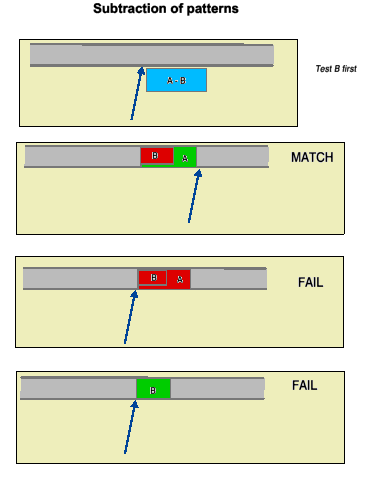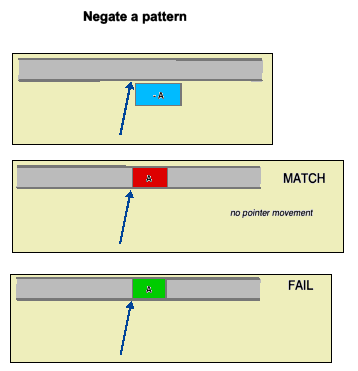Unary minus negates a pattern. So -A matches when A fails and fails when A matches. In both cases the pointer is not advanced. The pattern #A is equivalent to -(-A). It behaves just like A except that the pointer is not advanced.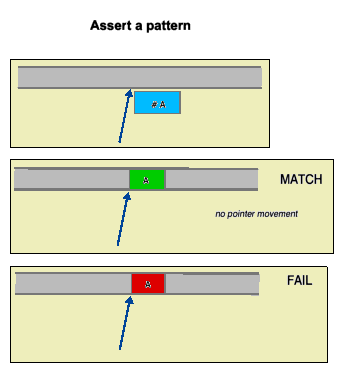We have not so far given any examples of actual patterns. For the details see the lpeg documentation. Bear in mind that because functions in Lua are not wedded to a single name, it makes sense to define local variables for all the lpeg functions one is going to use. So, for example, if the functions lpeg.match, lpeg.P and lpeg.C are to be the only ones from the lpeg library to be used in your script, we could write the script in a format like:

``` do
local P,C in lpeg
........ define a pattern here
local result = pattern:match(text)
........ output the result here
end

```
so that you can use the abbreviations P,C in place of lpeg.P,lpeg.C in defining your pattern.

The function lpeg.P converts values of other types into patterns. For example, it converts a string into the pattern that matches just that string, and a positive number n into the pattern that matches any n characters. The parser, when it sees that one of the operands of *,+ and - is a pattern, will automatically convert the other into a pattern using lpeg.P. This makes for a more abbreviated notation. For example, if x is a pattern, the pattern (x+1)^1 can be interpreted as x, possibly somewhere further on. We can interpret the expression as look for x, and, if it is not there, move the pointer by one character and repeat.

### Grammars

A grammar is essentially a set of equations, possibly recursive, for defining a pattern. Let us look at an example: bracketed expressions.

In the jargon of grammars we define two non-terminal symbols , E for expressions and B for bracketed expressions. Every expression is to be either a variable (a single lowercase letter) or a bracketed expression. Every bracketed expression is to be a pair of expressions inside parentheses. In the traditional notation for grammars we might write this as

```E -> var | B
B -> "(" E E ")"

```
Here is a script for it:

```      do
io.input(arg)
io.input()
local P,R,V in lpeg
local bra,ket = P "(",P ")"
local var = R "az"
local grammar = P { "E",
E = var + V"B";
B = bra*V"E"*V"E"*ket;
}
local ok = grammar:match(text)
local fmt = "%s is OK"
print(ok and fmt:format(text) or "FAIL")
end

```
So grammars are represented by tables. The primary nonterminal, E in our case, is quoted first. Then come the equations. Note that non-terminals occurring on the right hand side are quoted as arguments to the function lpeg.V.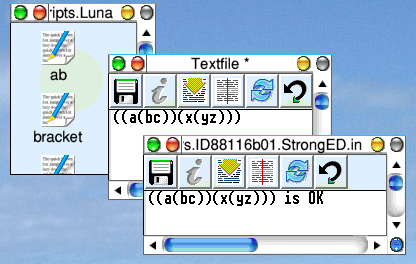Here is a picture showing the result of CTRL-dragging the script above onto the apply icon of a text file.

But is not the traditional notation for grammars simpler? Yes, but it cannot express the actions that we may want to endow our patterns with. The picture we have presented, of the three elements text, pointer, pattern is, as we warned, too simple. We also have to take into account captures. These are data accumulated from the text as subpatterns sweep over it, which a pattern can manipulate. The basic captures are either values of the pointer (where did a pattern match?), substrings (what did the pattern match?) or constant data that have nothing to do with the text. These basic captures can be transformed into complex captures by the application of functions or by being put into a table.

By way of an example let us modify the bracketed expression script above so that it evaluates the expression. For simplicity we suppose that what we termed variables just evaluate to themselves and that juxtaposition of two variables is to denote some binary operation on them; we take this to be the replacement of xy by the expression y[x].

```      do
io.input(arg)
io.input()
local P,R,V,C in lpeg
local bra,ket = P "(",P ")"
local var = R "az"
local s = "%s[%s]"
local f = \(x,y) => s:format(y,x) end
local grammar = P { "E",
E = C(var) + V"B";
B = (bra*V"E"*V"E"*ket)/f;
}
local ok = grammar:match(text)
print(ok or "FAIL")
end

```
There are two changes to the grammar: the adornment of var so that it captures substrings, and the adornment of the expression for the non-terminal B so that the two captures made by each V"E" are combined by the function f. The output is changed to show the final capture.

An input (a((bc)d))) produces an output d[c[b]][a].